## Factoring

To factor means to find two or more quantities whose product equals the original quantity.

#### Factoring out a common factor

To factor out a common factor, (1) find the largest common monomial factor of each term and (2) divide the original polynomial by this factor to obtain the second factor. The second factor will be a polynomial.

##### Example 1

Factor.

1. 5 x2 + 4 x = x(5 x + 4)

2. 2 y3 – 6 y = 2 y( y2 – 3)

3. x5 – 4 x 3 + x2 = x2( x3 – 4 x + 1)

When the common monomial factor is the last term, 1 is used as a place holder in the second factor.

#### Factoring the difference between two squares

To factor the difference between two squares, (1) find the square root of the first term and the square root of the second term and (2) express your answer as the product of the sum of the quantities from Step 1 times the difference of those quantities.

##### Example 2

Factor.

1. x2 – 144 = ( x + 12)( x – 12)

Note: x2 + 144 is not factorable.

2. a2b2 = ( a + b)( ab)

3. 9 y2 – 1 = (3 y + 1)(3 y – 1)

#### Factoring polynomials having three terms of the form ax2 + bx + c

To factor polynomials having three terms of the form ax 2 + bx + c, (1) check to see whether you can monomial factor (factor out common terms). Then if a = 1 (that is, the first term is simply x 2), use double parentheses and factor the first term. Place these factors in the left sides of the parentheses. For example,

( x )( x )

(2) Factor the last term and place the factors in the right sides of the parentheses.

To decide on the signs of the numbers, do the following. If the sign of the last term is negative, (1) find two numbers (one will be a positive number and the other a negative number) whose product is the last term and whose difference is the coefficient (number in front) of the middle term and (2) give the larger of these two numbers the sign of the middle term and the opposite sign to the other factor.

If the sign of the last term is positive, (1) find two numbers (both will be positive or both will be negative) whose product is the last term and whose sum is the coefficient of the middle term and (2) give both factors the sign of the middle term.

##### Example 3

Factor x2 – 3 x – 10.

First check to see whether you can monomial factor (factor out common terms). Because this is not possible, use double parentheses and factor the first term as follows: ( x ) ( x ). Next, factor the last term, 10, into 2 times 5 (5 must take the negative sign and 2 must take the positive sign because they will then total the coefficient of the middle term, which is –3) and add the proper signs, leaving

( x – 5)( x + 2)

Multiply means (inner terms) and extremes (outer terms) to check.To completely check, multiply the factors together.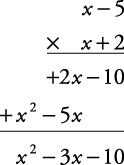##### Example 4

Factor x2 + 8 x + 15.

( x + 3)( x + 5)

Notice that 3 × 5 = 15 and 3 + 5 = 8, the coefficient of the middle term. Also note that the signs of both factors are +, the sign of the middle term. To check,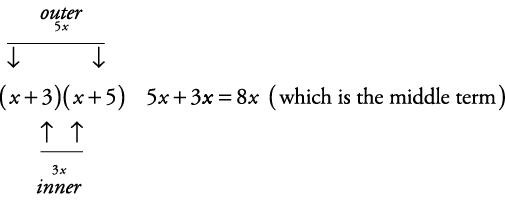##### Example 5

Factor x2 – 5 x – 14.

( x – 7)( x + 2)

Notice that 7 × 2 = 14 and 7 – 2 = 5, the coefficient of the middle term. Also note that the sign of the larger factor, 7, is –, while the other factor, 2, has a + sign. To check,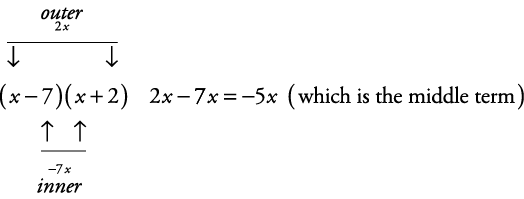If, however, a ≠ 1 (that is, the first term has a coefficient—for example, 4 x2 +5 x + 1), then additional trial and error will be necessary.

##### Example 6

Factor 4 x2 +5 x + 1.

(2 x + )(2 x + ) might work for the first term. But when 1s are used as factors to get the last term, (2 x + 1)(2 x + 1), the middle term comes out as 4 x instead of 5 x.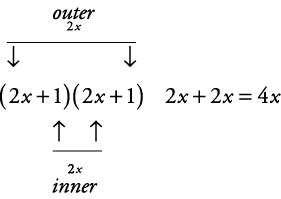Therefore, try (4 x + )( x + ). Now using 1s as factors to get the last terms gives (4 x + 1)( x + 1). Checking for the middle term,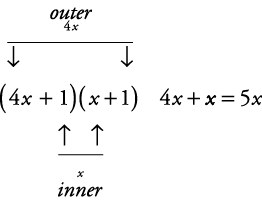Therefore, 4 x2 + 5 x + 1 = (4 x + 1)( x + 1).

##### Example 7

Factor 4 a2 + 6 a + 2.

Factoring out a 2 leaves

2(2 a2 + 3 a + 1)

Now factor as usual, giving

2(2 a + 1)( a + 1)

To check,##### Example 8

Factor 5 x3 + 6 x2 + x.

Factoring out an x leaves

x(5 x2 + 6 x + 1)

Now factor as usual, giving

x(5 x + 1)( x + 1)

To check,##### Example 9

Factor 5 + 7 b + 2 b2 (a slight twist).

(5 + 2 b)(1 + b)

To check,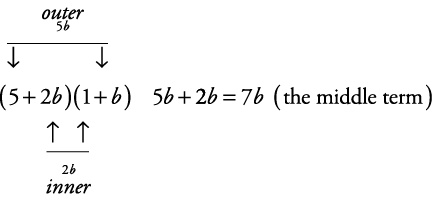Note that (5 + b)(1 + 2 b) is incorrect because it gives the wrong middle term.

##### Example 10

Factor x2 + 2 xy + y2.

( x + y)( x + y)

To check,##### Example 11

Factor 3 x2 – 48.

Factoring out a 3 leaves

3( x2 – 16)

But x2 – 16 is the difference between two squares and can be further factored into ( x + 4)( x – 4). Therefore, when completely factored, 3 x2 – 48 = 3( x + 4)( x – 4).

#### Factoring by grouping

Some polynomials have binomial, trinomial, and other polynomial factors.

##### Example 12

Factor x + 2 + xy + 2 y.

Since there is no monomial factor, you should attempt rearranging the terms and looking for binomial factors.

x + 2 + xy + 2 y = x + xy + 2 + 2 y

Grouping gives

( x + xy) + (2 + 2 y)

Now factoring gives

x(1 + y) + 2(1 + y)

Using the distributive property gives

( x + 2)(1 + y)

You could rearrange them differently, but you would still come up with the same factoring.

#### Summary of the factoring methods

When factoring polynomials, you should look for factoring in the following order.

1. Look for the greatest common factor if one exists.

2. If there are two terms, look for the difference of square numbers.

3. If there are three terms, look for a pattern that applies to ax2 + bx + c.

4. If there are four or more terms, look for some type of regrouping that will lead to other factoring.

Note: There are polynomials that are not factorable.

##### Example 13

Factor 2 x2 + 3 x + 5.

1. This polynomial does not have a common factor.

2. This polynomial is not a difference of square numbers.

3. There is no (_ x )(_ x ) combination that produces 2 x2 + 3 x + 5.

4. Since there are only three terms, there is no regrouping possibility.

Therefore, this polynomial is not factorable.